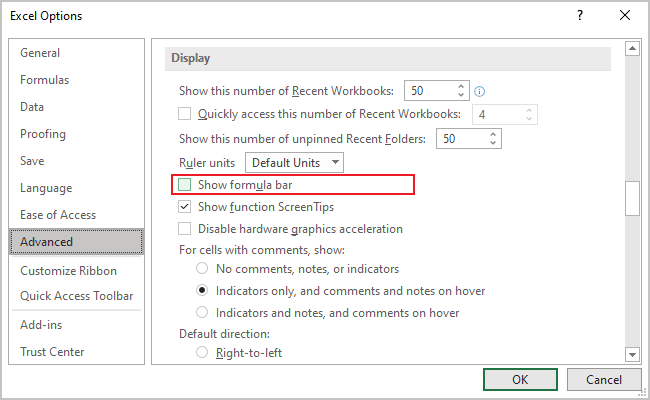# How to hide, display, and expand the Formula bar

Excel 365
The Formula bar displays data and formulas for the current cell in Microsoft Excel. You can expand the formula editor if the data or formula does not fit and hide the formula bar if you need more screen space.

## Expand and collapse the Formula bar

When working with long formulas in the default position of the Formula bar, you may not see the entire formula. Excel starts scrolling the text to the left as you continue typing. The best way to avoid this and see the whole formula is to expand the Formula bar. There are two ways to expand the formula editor:

• Drag the bottom edge of the Formula bar downward: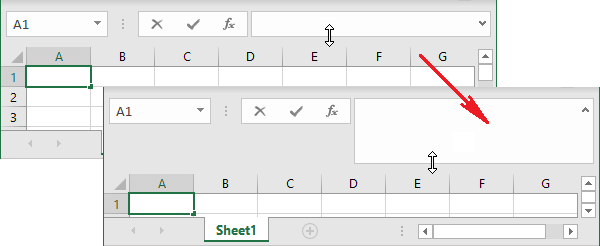• Click the arrow at the right corner of the Formula bar: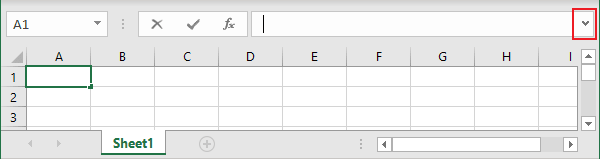Note: Press Ctrl+Shift+U to change the height of the Formula bar to display one row or the previous size.

## Hide the Formula bar

Many Excel users do not use the Formula bar but need more screen space (see how to collapse the Ribbon to get more space on the screen and hide spreadsheet tabs).

To hide the Formula bar, do one of the following:

• On the View tab, in the Show group, uncheck the Formula bar option: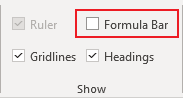• On the File tab, click the Options button: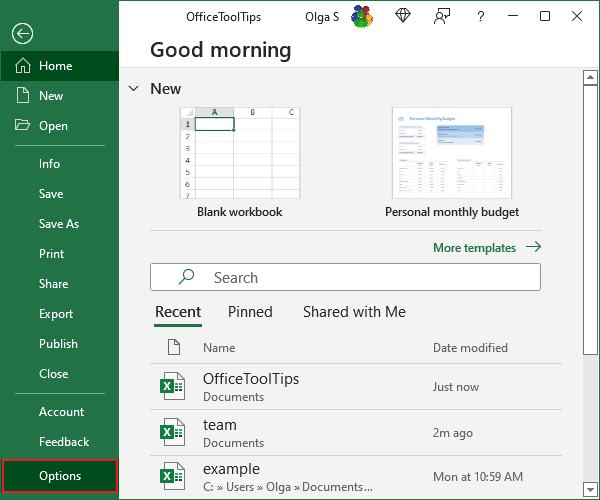In the Excel Options dialog box, in the Advanced tab, under Display, uncheck the Show formula bar option: